Next: Triatomic Molecule Up: Coupled Oscillations Previous: Normal Coordinates

Spring-Coupled Masses

Consider the two degree of freedom dynamical system pictured in Figure 37. In this system, two point objects of mass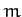are free to move in one dimension. Furthermore, the masses are connected together by a spring of spring constant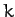, and are also each attached to fixed supports via springs of spring constant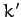.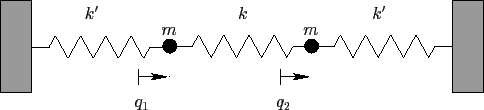Let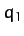and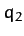be the displacements of the first and second masses, respectively, from the equilibrium state. It follows that the extensions of the left-hand, middle, and right-hand springs are,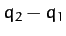, and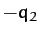, respectively. The kinetic energy of the system takes the form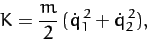(819)

whereas the potential energy is written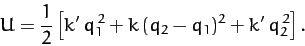(820)

The above expression can be rearranged to give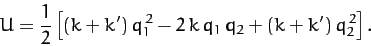(821)

A comparison of Equations (819) and (821) with the standard forms (775) and (780) yields the following expressions for the mass matrix,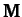, and the force matrix,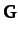: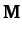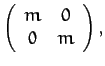(822)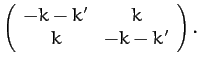(823)

Now, the equation of motion of the system takes the form [see Equation (786)]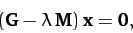(824)

whereis the column vector of theandvalues. The solubility condition for the above equation is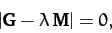(825)

or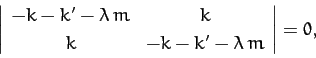(826)

which yields the following quadratic equation for the eigenvalue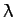: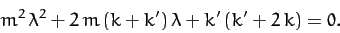(827)

The two roots of the above equation are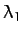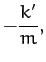(828)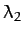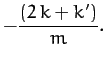(829)

The fact that the roots are negative implies that both normal modes are oscillatory in nature: i.e., the original equilibrium is stable. The characteristic oscillation frequencies of the modes are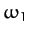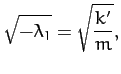(830)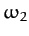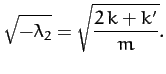(831)

Now, the first row of Equation (824) gives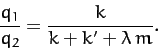(832)

Moreover, Equations (799) and (822) yield the following normalization condition for the eigenvectors: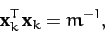(833)

for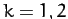. It follows that the two eigenvectors are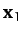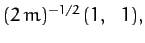(834)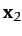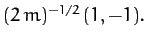(835)

According to Equations (830)-(831) and (834)-(835), our two degree of freedom system possesses two normal modes. The first mode oscillates at the frequency, and is a purely symmetric mode: i.e.,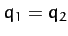. Note that such a mode does not stretch the middle spring. Hence,is independent of. In fact,is simply the characteristic oscillation frequency of a masson the end of a spring of spring constant. The second mode oscillates at the frequency, and is a purely anti-symmetric mode: i.e.,. Since such a mode stretches the middle spring, the second mode experiences a greater restoring force than the first, and hence has a higher oscillation frequency: i.e.,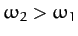.

Note, finally, from Equations (809) and (822), that the normal coordinates of the system are: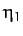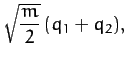(836)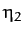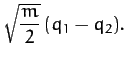(837)

When expressed in terms of these normal coordinates, the kinetic and potential energies of the system reduce to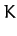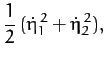(838)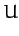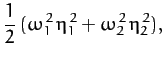(839)

respectively.Next: Triatomic Molecule Up: Coupled Oscillations Previous: Normal Coordinates
Richard Fitzpatrick 2011-03-31